# 【直播】我的基因组（20）:覆盖度详细探究

samtools flagstat P_jmzeng.final.bam

8597742 + 0 secondary

0 + 0 supplementary

132556557 + 0 duplicates

890858540 + 0 mapped (99.05% : N/A)

890764006 + 0 paired in sequencing

853255862 + 0 properly paired (95.79% : N/A)

881249604 + 0 with itself and mate mapped

1011194 + 0 singletons (0.11% : N/A)

20382234 + 0 with mate mapped to a different chr

12511988 + 0 with mate mapped to a different chr (mapQ>=5)

samtools mpileup P_jmzeng.final.bam |perl -alne '{if(\$F>100){\$depth{"over100"}++}else{\$depth{\$F}++}}END{print "\$_\t\$depth{\$_}" foreach sort{\$a <=> \$b}keys %depth}' 当是需要运行很久，毕竟全基因组的bam文件太大了。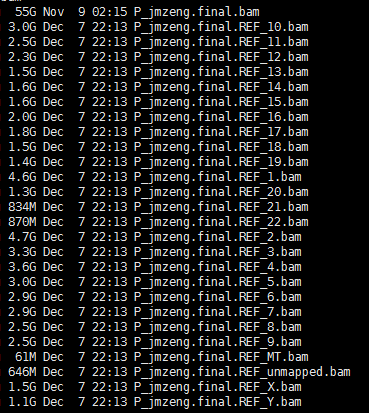do

echo \$id

samtools mpileup \$id |perl -alne '{if(\$F>100){\$depth{"over100"}++}else{\$depth{\$F}++}}END{print "\$_\t\$depth{\$_}" foreach sort{\$a <=> \$b}keys %depth}' >\$id.depth.txt

done

over100 110789

0 27065

1 1730286

2 2219409

3 2728526

4 3251046

5 3774335

6 4303971

~~~~~~~~~~~~~~~~~~~~~~~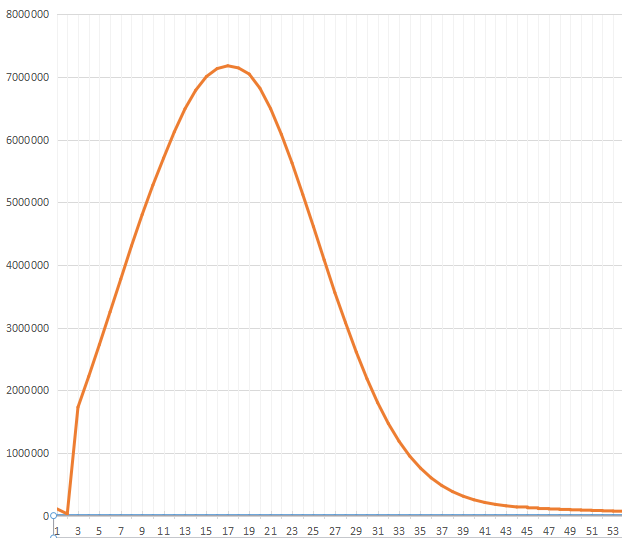plot(a[,2],type = 'l',xaxt="n",lwd=3)

axis(1, at = 1:nrow(a),labels =a[,1] , las=1)

abline(v=44)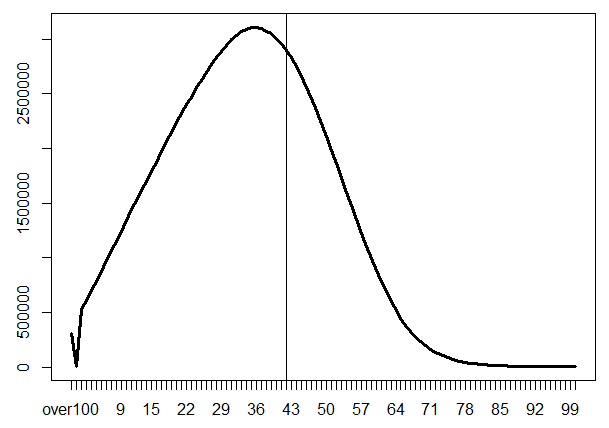over100=a[1,]

a=a[-1,]

a=rbind(a,over100)

a[,3]= cumsum(a[,2])

a[,4]= 1-a[,3]/sum(a[,2])

plot(a[,4],type = 'l',xaxt="n",lwd=3)

axis(1, at = 1:nrow(a),labels =a[,1] , las=1)

abline(v=10);abline(v=20);abline(v=30)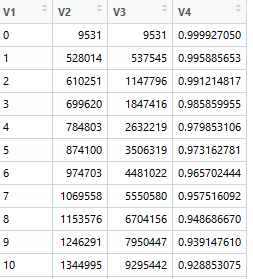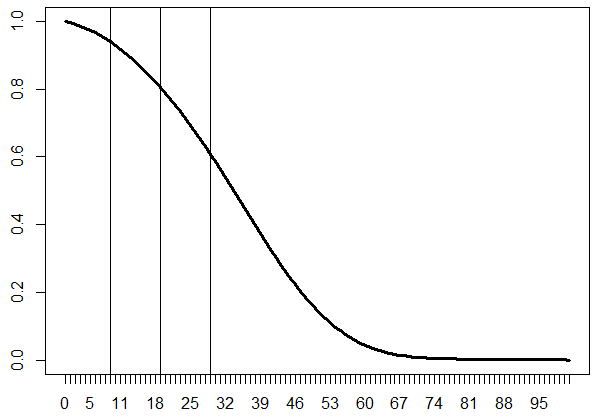https://www.biostars.org/p/104063/

http://www.gettinggeneticsdone.com/2014/03/visualize-coverage-exome-targeted-ngs-bedtools.html